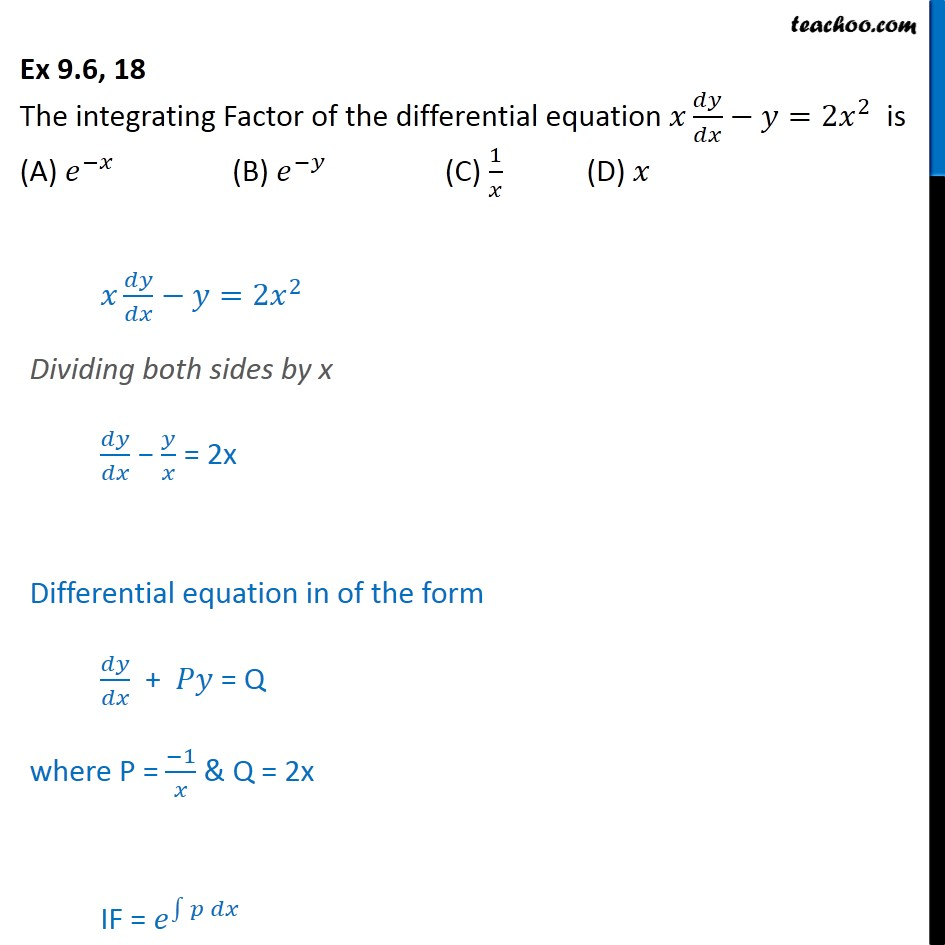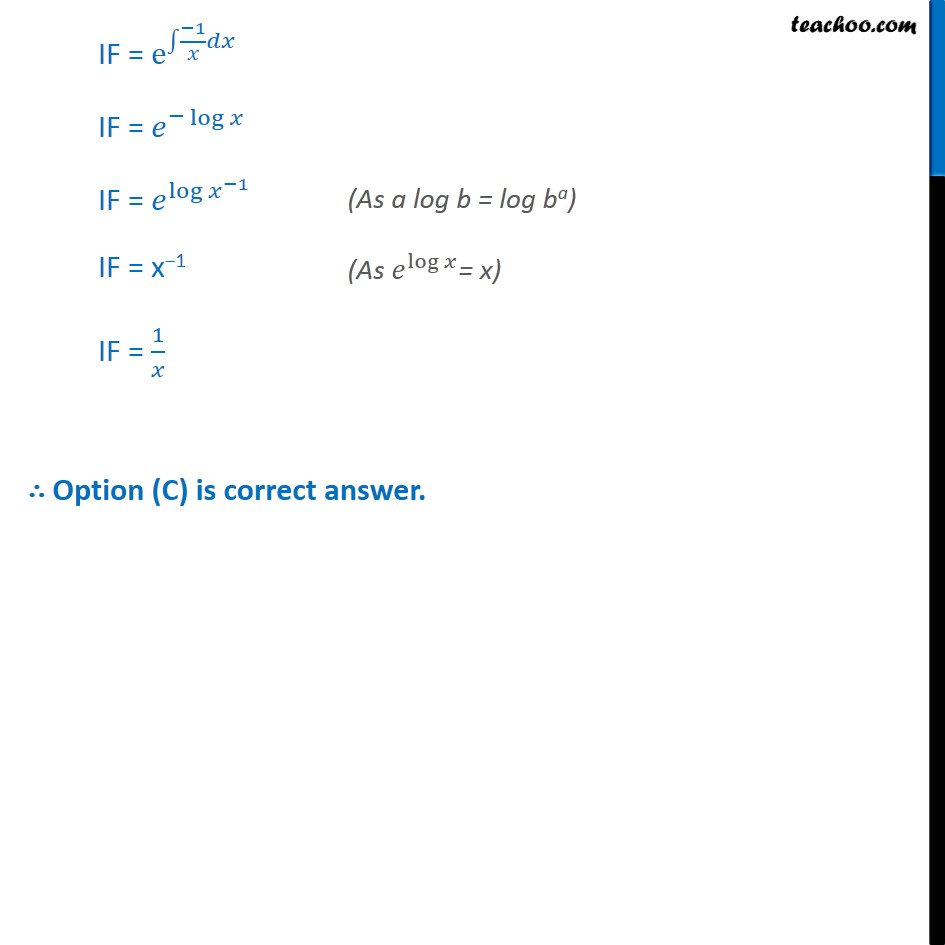Solving Linear differential equations - Equation given

Chapter 9 Class 12 Differential Equations
Concept wiseIntroducing your new favourite teacher - Teachoo Black, at only ₹83 per month

### Transcript

Ex 9.6, 18 The integrating Factor of the differential equation =2 2 is (A) (B) (C) 1 (D) =2 2 Dividing both sides by x = 2x Differential equation in of the form + = Q where P = 1 & Q = 2x IF = IF = e 1 IF = log IF = log 1 IF = x 1 IF = 1 Option (C) is correct answer.